# MCQs on Power Electronics

##### Page 6 of 10. Go to page 1 2 3 4 5 6 7 8 9 10
01․ SCRs are used in series to meet
high current demand.
low voltage demand.
low current demand.
high voltage demand.

In a series connection voltage division takes place but current remain same through over the line.

02․ To meet high current demand we use SCRs in
series connection.
parallel connection.
anti parallel connection.
both B and C.

Current division takes place in case of parallel connection but in anti parallel connection desired current division does not take place as SCRs.

03․ By which one of the following we can measure the reliability of a string?
String efficient.
Reliability factor.
Factor of safety.
Derating factor.

Derating factor (DRF) is used to measure the reliability of a string which given by, DRF = 1 - String efficiency.

04․ Calculate the maximum voltage that can be safely blocked by the string shown in figure, if maximum allowable leakage current is 2 mA?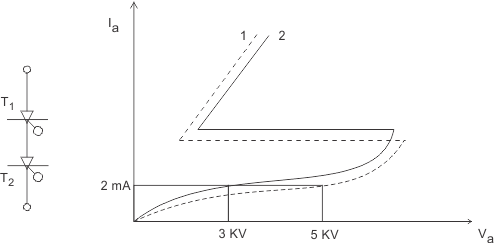10 KV.
6 KV.
8 KV.
2 KV.

In series connection same leakage current flows through all SCRs. Now SCRs have difference in their I-V characteristics. So unequal volume division will take place. Here volume across T1 and T2 is 5 KV and 3 KV respectively. So, maximum 5 + 3 = 8 KV can be safely applied.

05․ A thyristor string is made of a no. of SCR connected in series and parallel. The string have volume and current of 11 KV and 4 KA. The voltage and current rating of available SCRs are 1800 V and 1000 A. For a string efficiency of 90 % let the number of SCRs in series and parallel are a and b respectively. Then the value of a and b will be
5, 7.
4, 6.
7, 5.
6, 4.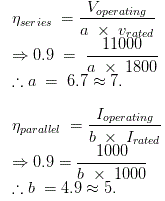06․ 60 thyrsistors are connected in series and parallel to form a 10 KV and 5.5 KA switch. Each thyristor is rated for 1.2 KV, 1 KA. The no. of parallel path are 6. The efficiency of the switch is
76.3 %.
91.6 %.
83.3 %.
90.9 %.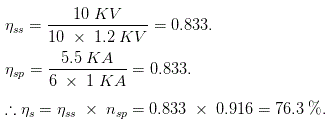07․ Figure shows two thyristors rated 400 A sharing a load current. Current through T2 is 180A. Current through T1 will be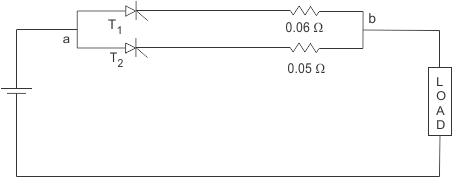100 A.
150 A.
120 A.
110 A.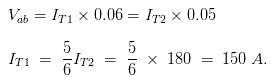08․ A 200 A thyristor is to be operated in parallel with a 300 A thyristor. The ON state voltage drops are 1.5 V and 1.2 Volts. What is the value of resistance R to be connected in series with each thyristor, so that current through the combination is 500 A and each of them is fully loaded ?
0.03 ohm
0.3 ohm
3.0 ohm
0.3 ohm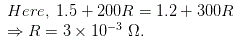09․ Dynamic equalizing circuit is used for
equal division of voltage across each thyristor.
equal division of current through each thyristor in parallel.
equal division of voltage across each thyristor in parallel.
equal division of current through each thyristor in series.

Dynamic equalizing circuit is nothing but snubber circuit which is used to equalize the voltage division under transient condition.

10․ 4 thyristors rated 200 V in series. The operating voltage of the string is 0.600 V. Derating factor of the string is
0.75.
0.7.
0.2.
0.25.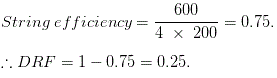<<<45678>>>